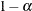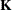# The SURVEYPHREG Procedure

### LSMESTIMATE Statement

• LSMESTIMATE model-effect <'label'> values <divisor=n> <, …<'label'> values <divisor=n>></ options>;

The LSMESTIMATE statement provides a mechanism for obtaining custom hypothesis tests among least squares means.

Table 113.5 summarizes the options available in the LSMESTIMATE statement.

Table 113.5: LSMESTIMATE Statement Options

Option

Description

Construction and Computation of LS-Means

Modifies covariate values in computing LS-means

Computes separate margins

Specifies a list of values to divide the coefficients

Specifies the weighting scheme for LS-means computation as determined by a data set

Tunes estimability checking

Degrees of Freedom and p-values

Determines the method for multiple-comparison adjustment of LS-means differences

ALPHA=Determines the confidence level ()

Performs one-sided, lower-tailed inference

Adjusts multiple-comparison p-values further in a step-down fashion

Specifies values under the null hypothesis for tests

Performs one-sided, upper-tailed inference

Statistical Output

Constructs confidence limits for means and mean differences

Displays the correlation matrix of LS-means

Displays the covariance matrix of LS-means

Prints thematrix

Prints thematrix

Produces a joint F or chi-square test for the LS-means and LS-means differences

Requests graphs of means and mean comparisons

Specifies the seed for computations that depend on random numbers

Generalized Linear Modeling

Specifies how to construct estimable functions with multinomial data

Exponentiates and displays LS-means estimates

Computes and displays estimates and standard errors of LS-means (but not differences) on the inverse linked scale

For details about the syntax of the LSMESTIMATE statement, see the section LSMESTIMATE Statement in Chapter 19: Shared Concepts and Topics.